Electro-Magnetic Interference MCQ Level - 1

# Electro-Magnetic Interference MCQ Level - 1

Test Description

## 25 Questions MCQ Test | Electro-Magnetic Interference MCQ Level - 1

Electro-Magnetic Interference MCQ Level - 1 for Class 12 2023 is part of Class 12 preparation. The Electro-Magnetic Interference MCQ Level - 1 questions and answers have been prepared according to the Class 12 exam syllabus.The Electro-Magnetic Interference MCQ Level - 1 MCQs are made for Class 12 2023 Exam. Find important definitions, questions, notes, meanings, examples, exercises, MCQs and online tests for Electro-Magnetic Interference MCQ Level - 1 below.
Solutions of Electro-Magnetic Interference MCQ Level - 1 questions in English are available as part of our course for Class 12 & Electro-Magnetic Interference MCQ Level - 1 solutions in Hindi for Class 12 course. Download more important topics, notes, lectures and mock test series for Class 12 Exam by signing up for free. Attempt Electro-Magnetic Interference MCQ Level - 1 | 25 questions in 25 minutes | Mock test for Class 12 preparation | Free important questions MCQ to study for Class 12 Exam | Download free PDF with solutions
 1 Crore+ students have signed up on EduRev. Have you?
Electro-Magnetic Interference MCQ Level - 1 - Question 1

### The energy stored in an inductor of self inductance Lhenry carrying a current of 1 A is

Electro-Magnetic Interference MCQ Level - 1 - Question 2

### A rectangular loop has a sliding connector PQ of lengthI and resistance RΩ and it is moving with a speed v asshown. The set-up is placed in a uniform magnetic fieldgoing into the plane of the paper. The three currents I1, I2, I are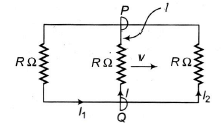Electro-Magnetic Interference MCQ Level - 1 - Question 3

### When a rod of length I is rotated with angular velocityof w in a perpendicular field of induction B, about oneend, the emf across its ends is

Electro-Magnetic Interference MCQ Level - 1 - Question 4

The oscillating frequency of a cyclotron is 10 MHz. Ifthe radius of its dees is 0.5m, the kinetic energy of aproton, which is accelerated by the cyclotron is

Electro-Magnetic Interference MCQ Level - 1 - Question 5

According to phenomenon of mutual inductance

Electro-Magnetic Interference MCQ Level - 1 - Question 6

A small square loop of wire of side I is placed inside alarge square loop of side L (L>>I). If the loops arecoplanar and their centres coincide, the mutual inductionof the system is directly proportional to

Electro-Magnetic Interference MCQ Level - 1 - Question 7

A rod PQ is connected to the capacitor plates. The rodis placed in a magnetic field (B) directed downwardsperpendicular to the plane of the paper. If the rod ispulled out of magnetic field with velocity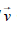as shown in Fig.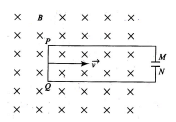Electro-Magnetic Interference MCQ Level - 1 - Question 8

A flexible wire bent in the form of a circle is place in a uniform magnetic field perpendicularly to the plane of the coil. The radius of the coil changes as shown in Fig. The graph of magnitude of induced emf in the coil is represented by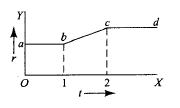Electro-Magnetic Interference MCQ Level - 1 - Question 9

A thin circular ring of area A is perpendicular to uniformmagnetic field of induction B. A small cut is made inthe ring and a galvanometer is connected across theends such that the total resistance of circuit is R. Whenthe ring is suddenly squeezed to zero area, the chargeflowing through the galvanometer is

Electro-Magnetic Interference MCQ Level - 1 - Question 10

A conducting wire xy of lengthi and mass m is slidingwithout friction on vertical conduction rails ab and cdas shown in Fig. A uniform magnetic field B exists perpendicular to the plane of the rails, x moves with aconstant velocity of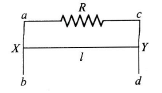Electro-Magnetic Interference MCQ Level - 1 - Question 11

A conducting wire of mass m slides down two smooth conducting bars, set at an angle q to the horizontal as shown in Fig. The separation between the bars isi .The system is located in the magnetic field B,perpendicular to the plane of the sliding wire and bars.The constant velocity of the wire is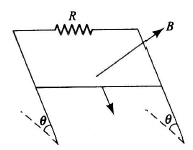Electro-Magnetic Interference MCQ Level - 1 - Question 12

Magnetic flux linked with a stationary loop of resistanceR varies with respect to time during the time period Tas follows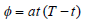The amount of heat generated in the loop during that time (inductance of the coil is negligible) is

Electro-Magnetic Interference MCQ Level - 1 - Question 13

An elasticized conducting band is around a sphericalballon. Its plane passes through the centre of theballoon. A uniform magnetic field of magnitude 0.04 T isdirected perpendicular to the plane of the band. Air islet one of the ballon at 100 cm3/s at an instant when the radius of the ballon is 10 cm. The induced emf inthe band is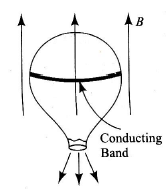Electro-Magnetic Interference MCQ Level - 1 - Question 14

A uniform magnetic field of induction B fills a cylindricalvolume of radius R,A rod AB of length 2i is placed asshown in Fig. If B is changing at the rate dB/dt, the emfthat is produced by the changing magnetic field andthat acts between the ends of the rod is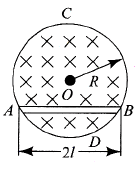Electro-Magnetic Interference MCQ Level - 1 - Question 15

Change Q is uniformly distributed on a thin insulatingring of mass m which is initially at rest. To what angularvelocity will the ring be accelerated when a magneticfield B, perpendicular to the plane of the ring, is switched on?

Electro-Magnetic Interference MCQ Level - 1 - Question 16

A vertical ring of radius r and resistance R falls vertically.it is in contact with two vertical rails which are joined atthe top. The rails are without friction and resistance.There is a horizontal uniform magnetic field of magnitudeB perpendicular to the plane of the ring and the rails.When the speed of the ring is v, the current in the tophorizontal of the rail section is

Electro-Magnetic Interference MCQ Level - 1 - Question 17

A metal disc of radius a rotates with a constant angular velocity w about its axis. The potential difference between the centre and the rim of the disc is (m =mass of electron, e = charge on electron)

Electro-Magnetic Interference MCQ Level - 1 - Question 18

The radius of the circular conducting loop shown in Fig.is R. Magnetic field is decreasing at a constantrate a .Resistance per unit length of the loop is ρ .Then, the current in wire AB is (AB is one of the diameters)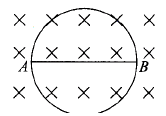Electro-Magnetic Interference MCQ Level - 1 - Question 19

A long conducting wire AH is moved over a conductingtriangular wire CDE with a constant velocity v in a uniformmagnetic field Bur directed into the plane of the paper.Resistance per unit length of each wire is ρ. Then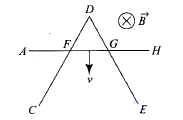Electro-Magnetic Interference MCQ Level - 1 - Question 20

A square coil ACDE with its plane vertical is releasedfrom rest in a horizontal uniform magnetic field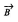of length 2 L (Fig). The acceleration of the coil is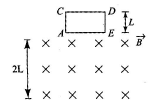Electro-Magnetic Interference MCQ Level - 1 - Question 21

The current through the coil in Fig. (i) varies as shown in Fig. (ii). Which graph best shows the ammeter A reading as a function of time?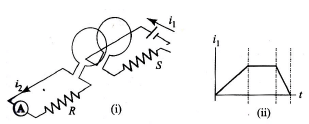Electro-Magnetic Interference MCQ Level - 1 - Question 22

In Fig. there is a conducting ring having resistance Rplaced in the plane of paper in a uniform magnetic field B0. If the ring is rotating in the plane of paper about anaxis passing through point O and perpendicular to theplane of paper with constant angular speed ω in clockwise direction, then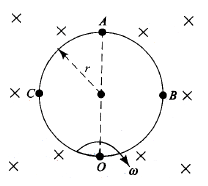Electro-Magnetic Interference MCQ Level - 1 - Question 23

There is a uniform magnetic field B in a circular regionof radius R as shown in Fig. Whose magnitude changesat the rate of dB/dt. The e.m.f. induced across the endsof a circular concentric conducting are of radius Rhaving an angle q as shown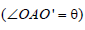is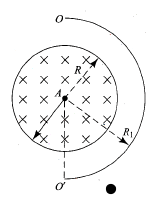Electro-Magnetic Interference MCQ Level - 1 - Question 24

In Fig. a square loop PQRS of side a and resistance ris placed near an infinitely long wire carrying a constantcurrent I. The sides PQ and RS are parallel to the wire.The wire and the loop are in the same plane. The loopis rotated by 1800 about an axis parallel to the long wireand passing through the mid-points of the sides QR and PS. The total amount of charge which passesthrough any point of the loop during rotation is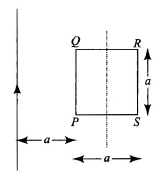Electro-Magnetic Interference MCQ Level - 1 - Question 25

Loop A of radius r << R moves towards loop B with aconstant velocity V in such a way that their planes arealways parallel. What is the distance between the two loops (x) when the induced emf in loop A is maximum?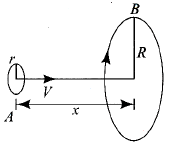Use Code STAYHOME200 and get INR 200 additional OFF Use Coupon Code
Information about Electro-Magnetic Interference MCQ Level - 1 Page
In this test you can find the Exam questions for Electro-Magnetic Interference MCQ Level - 1 solved & explained in the simplest way possible. Besides giving Questions and answers for Electro-Magnetic Interference MCQ Level - 1, EduRev gives you an ample number of Online tests for practice